Latest SSC jobs   »   Reasoning Quiz for RRB NTPC :...

# Reasoning Quiz for RRB NTPC : 17th February For Venn Diagram & Counting Figure

Q1. 7th of April 2014 is a Monday. What day would be 14 August 2014?
(a) Wednesday
(b) Friday
(c) Thursday
(d) Monday

Q2. In the following question select the related word from the given alternative?
GATES : JDWHV : : LINUX :
(a) OLQXA
(b) OLQVZ
(c) NKQXA
(d) NKQVZ

Q3. The given Venn diagram represents the sports preference of a group of school students. There are three games : A-Hockey, B-Cricket, C-Squash. How many student play at least one game?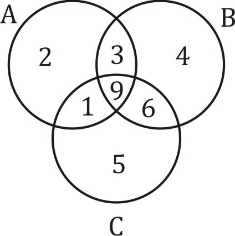(a) 15
(b) 21
(c) 30
(d) 25

Q4. Choose the figure which is different from the others in the given set.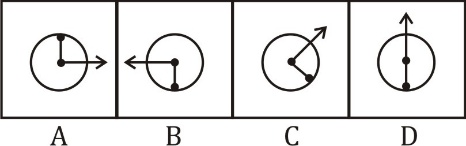(a) A
(b) B
(c) C
(d) D

Q5. Which of the following correctly represents the relationship between.
1. Eyes
3. Face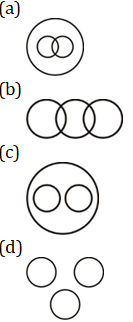Q6. Out of the four given figures, three are similar in a certain manner. However, one figure is NOT like the other three.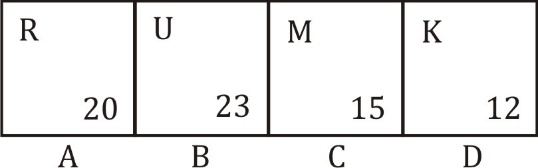(a) A
(b) B
(c) C
(d) D

Q7. Direction : There are statement(s) which are followed by conclusion(s). Choose the conclusion which logically follow from the given statements
Statement: Domestic demand has been increasing faster than the production of indigenous crude oil.
Conclusion:
I. Crude oil must be imported
II. Domestic demand should be reduced
(a) If only conclusion I follows;
(b) If only conclusion II follows;
(c) If either I or II follows;
(d) If neither I nor II follows; and

Q8. In a certain language, ‘MEI TOH CHALA’ is coded as ‘mo to yo’, ‘CHALA YE BHI’ is coded as ‘ao mo xo’, ‘MEI PAR TOH’ is coded as ‘ho yo to’, What is the code for ‘YE MEI BHI’ in that language?
(a) ao to xo
(b) ho to yo
(c) ao xo yo
(d) ‘ao xo yo’ OR ‘ao to xo’

Q9. How many triangles are there in the following figure?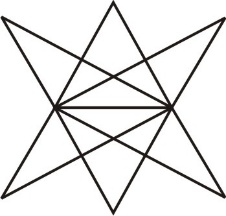(a) 16
(b) 18
(c) 22
(d) more than 23

Q10. Choose figure that is different from the rest.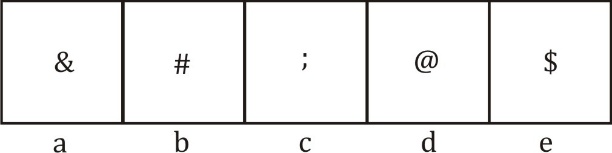(a) b
(b) c
(c) e
(d) a

#### Solutions:

S1. Ans.(c)
Sol.
7 April to 14 August = 23 + 31 + 30 + 31 + 14 = 129 days
129/7 = 3 odd days
So, 14 August = Monday + 3 = Thursday.

S2. Ans.(a)
Sol.
+3 pattern

S3. Ans.(c)
Sol.
No. of students = 30

S4. Ans.(d)
Sol.
Except (d), all other have a 90 degree angle between both hands.

S5. Ans.(c)
Sol.

S6. Ans.(d)
Sol.
R → 18 + 2 = 20
U → 21 + 2 = 23
M → 13 + 2 = 15
K → 11 + 2 = 13 ≠ 12

S7. Ans(c)
Sol.
The statement mentions that demand for oil is increasing faster than the production. So. Either the demand must be reduced or oil must be imported to cope with the increasing demand. Thus either I or II follows.

S8. Ans.(d)
Sol.
CHALA → mo
MEI/TOH → yo/to

S9. Ans.(d)
Sol.
No. of triangles = more than 23

S10. Ans.(b)
Sol.
Only figure ‘c’ is punctuation sign.

RRB NTPC Previous year Cut Off | 1st & 2nd Stage Examination
RRB NTPC Recruitment 2019: Check FAQs
RRB NTPC Exam Pattern 2019 – Check Here
RRB NTPC Previous Year Exam Analysis#### Congratulations!General Awareness & Science Capsule PDFIncorrect details? Fill the form again here

General Awareness & Science Capsule PDF

Thank You, Your details have been submitted we will get back to you.
•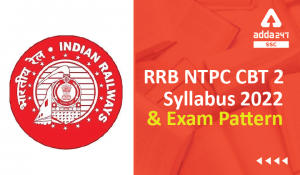RRB NTPC CBT 2 Syllabus 2022, Topics, Su...
•GA Most Scoring Topics for RRB NTPC CBT ...
•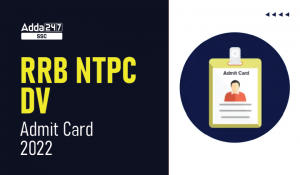RRB NTPC DV Admit Card 2022 Out, Downloa...
•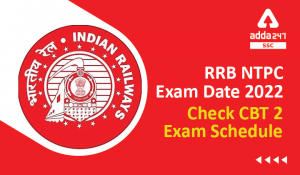RRB NTPC Document Verification 2022 | C...
•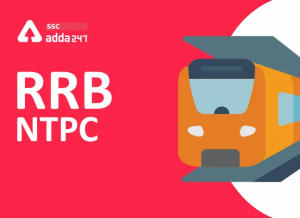RRB NTPC 2022 Notification, Exam Date, L...
•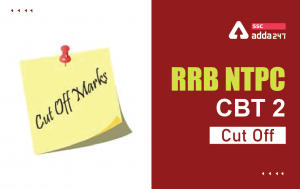RRB NTPC Cut Off 2022 Out for CBAT Exam,...